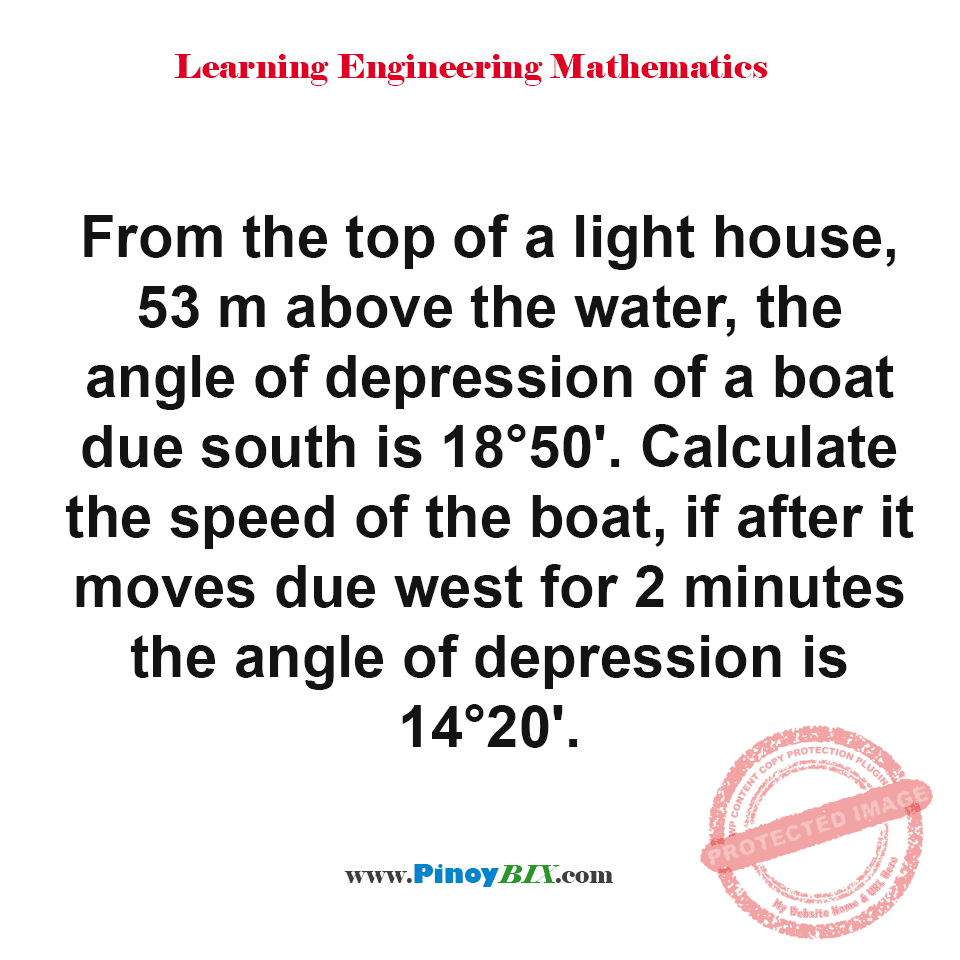# Solution: Calculate the speed of the boat

(Last Updated On: January 28, 2020)#### Problem Statement:

From the top of a light house, 53 m above the water, the angle of depression of a boat due south is 18°50′. Calculate the speed of the boat, if after it moves due west for 2 minutes the angle of depressions 14°20′.

The speed of the boat is 69.26 meters/min.

#### Latest Problem Solving in Plane Trigonometry Problems

More Questions in: Plane Trigonometry Problems